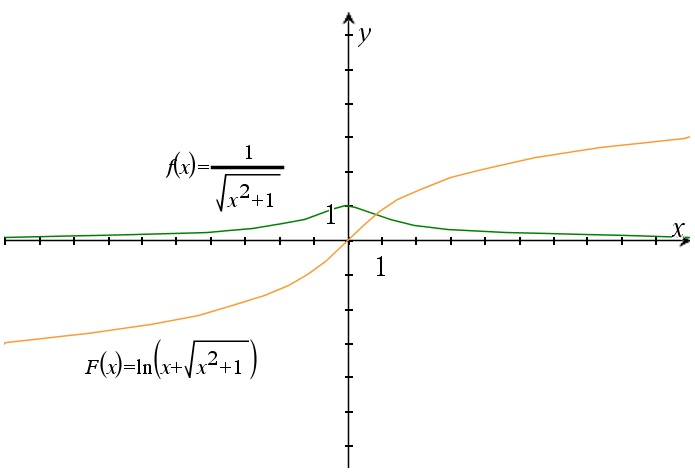# The integral of one over sqrt(x^2+1) dx

Up a level : Integrals
Previous page : The integral of 1/sqrt(x^2-1) dx
Next page : The integral of sqrt(1-x^2) dxLet us look at$\int {\frac{{dx}}{{\sqrt {{x^2} + 1} }}}$

Using the fact from the previous page we have that a=i, and thus that$\begin{gathered} \int {\frac{{dx}}{{\sqrt {{x^2} - {a^2}} }}} = \int {\frac{{dx}}{{\sqrt {{x^2} - {i^2}} }}} \hfill \\ \quad = \ln \left( {x + \sqrt {{x^2} - {i^2}} } \right) + C \hfill \\ \quad = \ln \left( {x + \sqrt {{x^2} + 1} } \right) + C \hfill \\ \end{gathered}$

This is also called the arsinh(x) or sinh-1(x) ( see this page). A graph of the integrand vs. the integral can be seen below.Up a level : Integrals
Previous page : The integral of 1/sqrt(x^2-1) dx
Next page : The integral of sqrt(1-x^2) dxLast modified: Feb 21, 2022 @ 15:14